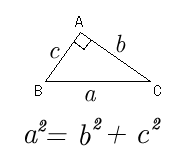Click on the link below for Really cool conversion factors
 Right Triangles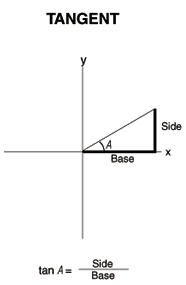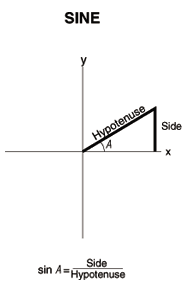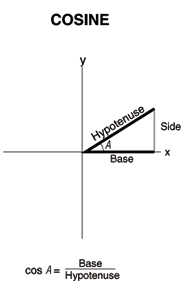Right Triangles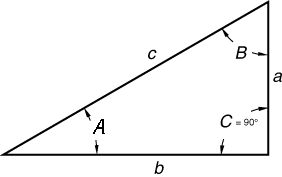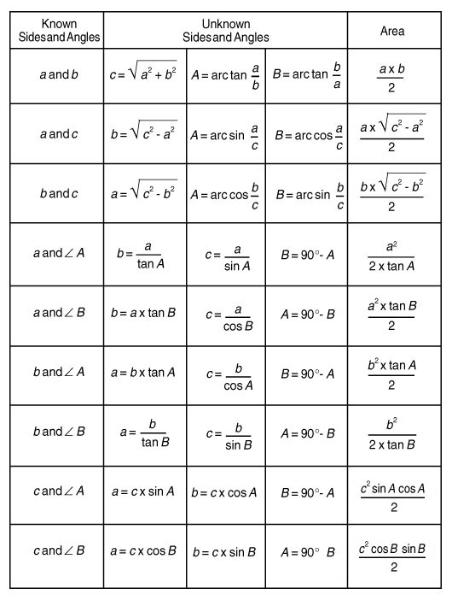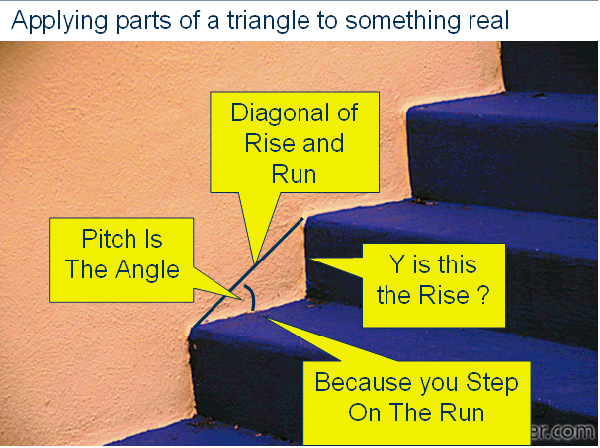Do You see the Triangle on the Stairs ?

Using the top row of keys on your ITI
Sheet Metal/HVAC Pro provides instant solutions in dimensional format
for Right Triangle problems for sheet metal problems.

A Right Triangle is calculated simply by entering two of four variables:

1)            (Run)

2)            (Rise)

3)            (Diagonal, or Hypotenuse); or

4)            (Theta/Pitch).

x=Run — Enters or calculates “x,” or the Run or horizontal leg (base) of a Right Triangle.

y=Rise — Enters or calculates “y,” or the Rise or vertical leg (height) of a Right Triangle.

r=Hypotenuse or Diagonal ---Enters or calculates the Hypotenuse or diagonal leg of a Right Triangle.

=Theta---Enters or calculates the Pitch (slope) of a roof (or Right Triangle).
Math Page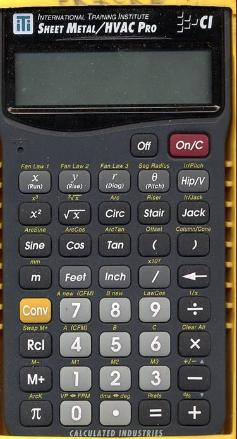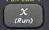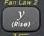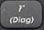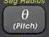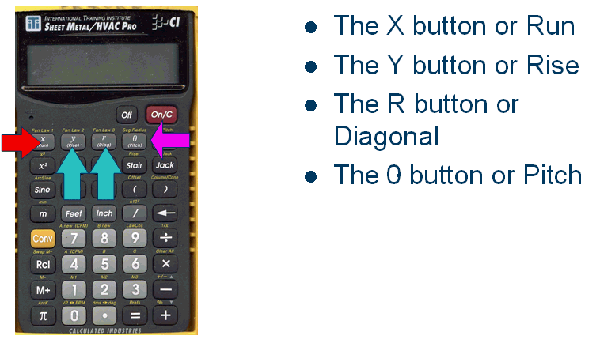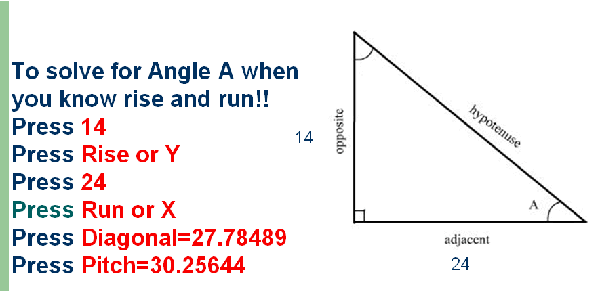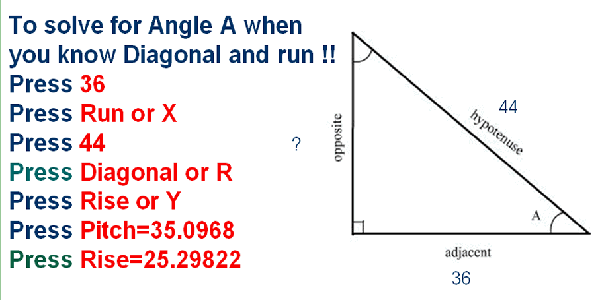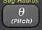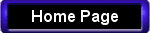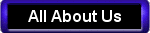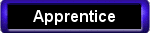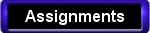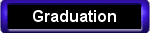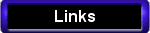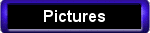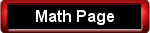Sine means the relationship between the side opposite or rise of a triangle and its Hypotenuse
 Cosine means the relationship between the side adjacent or run of a triangle and its Hypotenuse
 Tangent means the relationship between the side adjacent or run of a triangle and the side Opposite or rise of a triangle
Oppisite
Oppisite
Oppisite
 Pythagorean Theorem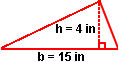TO FIND THE VOLUME OF A WEDGE1/2 TIMES ALTITUDE TIMES THE BASE TIME THE HEIGHT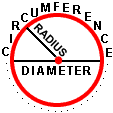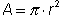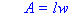*
 Area = Length times Width
 Area = Pi times the  radius squared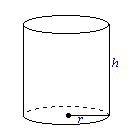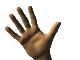There are five formula that every Apprentice will know by the time they graduate. Look for the hands that tell you which ones are on all of your upgrade tests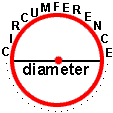TO FIND THE CIRCUMFERENCE OF A CIRCLEPI TIMES THE DIAMETER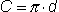Volume = Pi times the  radius squared times the Height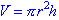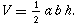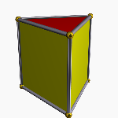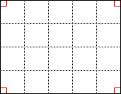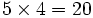Volume =Length Times Width Times Height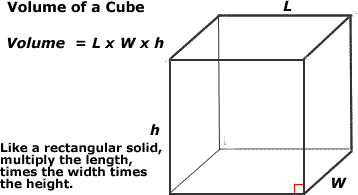AREA = 1/2 TIMES THE BASE TIMES THE HEIGHT    OR    BASE TIMES THE HEIGHT DIVIDED BY 2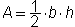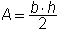OR